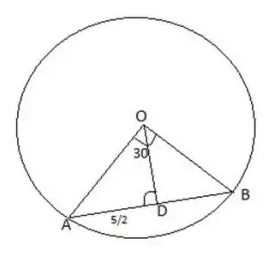# A chord of length 5 cm subtends an | Quantitative Aptitude – Geometry – Circles

### CAT Verbal Ability

CAT 2018 – The CAT QA section has grown increasingly difficult since 2015. In order to tackle the tougher CAT Level QA questions for the CAT Exam, it is important to understand the basics of CAT. To obtain a great CAT score, make use of MBAP Free Study material with detailed solutions and video explanations. Check out MBAP free Mock test to take these questions in a test format for free.

### You may also find remaining question solution of CAT 2018, Slot-2 by searching the question in the search bar.

The question below is from previous year CAT question from CAT 2018 exam comes from CAT Geometry – Circles – A chord of length 5 cm subtends an…. Find out by answering this question which tests an aspirant’s Quantitative Ability Skills:

## CAT 2018 – Slot -2 - Question 2 - A chord of length 5 cm subtends an

##### Q. 2: A chord of length 5 cm subtends an angle of 60° at the centre of a circle. The length, in cm, of a chord that subtends an angle of 120° at the centre of the same circle is
1. 8
2. 6√2
3. 5√3
4. 2In triangle ODA, OA=AD cosec 30=5
So if the angle AOB is 120 degree, then angle AOD will be 120/2=60
In the case length of chord = 2×OA Sin 60=5√3Counselling Session
By IIM Mentor

#### Free Material Area